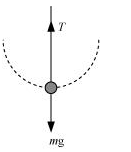## Pages

### Laws of motion NCERT Solutions Class 11 Physics - Solved Exercise Question 5.26

Question 5.26:
A stone of mass m tied to the end of a string revolves in a vertical circle of radius R. The net forces at the lowest and highest points of the circle directed vertically downwards are: [Choose the correct alternative]

 Lowest Point Highest Point (a) mg – T1 mg + T2 (b) mg + T1 mg – T2 (c) mg + T1 – (mv12) / R mg – T2 + (mv12) / R (d) mg – T1 – (mv12) / R mg + T2 + (mv12) / R

T1 and V1 denote the tension and speed at the lowest point. T2 and v2 denote corresponding values at the highest point.

Solution:
(a) The free body diagram of the stone at the lowest point is shown in the following figure.According to Newton’s second law of motion, the net force acting on the stone at this point is equal to the centripetal force, i.e.,
Fnet = T - mg = mv12 / R   ....(i)
Where, v1 = Velocity at the lowest point

The free body diagram of the stone at the highest point is shown in the following figure.Using Newton’s second law of motion, we have:
T + mg = mv22 / R    ...(ii)
Where, v2 = Velocity at the highest point
It is clear from equations (i) and (ii) that the net force acting at the lowest and the highest points are respectively (Tmg) and (T + mg).